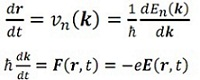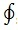#### Electrodynamics, Physics tutorial

Introduction:

Electrodynamics is a theory of fields, and all matter enters theory in form of densities. Every modern physical theory is field theories, from general relativity to quantum fields in Standard Model and string theory.

It is a branch of physics which deals with effects arising from interactions of electric currents with magnets, with other currents, or with themselves

Electrodynamics:

Given the functions En(k) the semi-classical model relates with each electron a position, a wave vector and a band index  'n'. In the presence of applied fields the position, the wave vector, and the index are taken to evolve according to the given rules:

1) The band index is a constant of the motion. The semi-classical model avoids the chance of interband transitions. This means that in this model it supposed that the applied electric field is small.

2) The time advancement of the position and the wave-vector of an electron having band index 'n' are determined via the equations of motion:Maxwell's equations:

Electric and magnetic fields are generated by charges, either at rest or in motion. Such charges satisfy significant law of charge conservation. This is a law which appears to be severely satisfied in nature. Carriers of electric charge, at microscopic level these are elementary particles, may be produced and may disappear, but in these processes total charge is always preserved.

With Q as total electric charge within given volume, charge conservation may be expressed as

dQ/dt

Though, this equation is correct only when there is no charge passing through boundary surface of selected volume. More general expression is thus

dQ/dt = -I

Here I is current through the boundary surface.

In general, when motion of charged particles can be explained by (smooth) velocity field v(r, t), we have the following relation between current and charge densities

j(r,t) = v(r,t)Ρ(r,t)

This law states how electric charge serves as source for electric field. As all of electromagnetic equations it can be given integral or differential form. In integral form it relates flux of electric field through any given closed surface S to total charge Q within surface

sE.dS = Q/ε0

Where ε0 is permittivity of vacuum, with value ε0 = 8.85.10-12C2/Nm2

Gauss' law is fundamental equation of electrostatics, where basic problem is to find out electric field from given charge distribution, with specified boundary conditions satisfied by field. In its simplest form problem is to find out field from isolated point charge, in which case Gauss' law in integral form can easily be solved under assumption of rotational symmetry.

Ampere's law:

This law states how electric currents generate magnetic field and it illustrates that line integral of magnetic field around any closed curve C gets two contributions, one from total electric current I passing through C and other from displacement current, that is stated by time derivative of electric flux through surface S with C as boundary. Integral form iscB.ds = μ0I + (1/c2)(d/dt) ∫sE.ds

Gauss' law for magnetic field and Faraday's law of induction:

The significant property of magnetic field is that there exists no isolated magnetic pole. This signifies that total magnetic flux through any closed surface S vanishes. Total magnetic flux through any closed surface S is zero.

Thus magnetic flux lines have no end points.

sB.ds = 0 and in differential form this gives ∇.B = 0

It has similar form as Gauss' law for electric field, but in this situation there is no counterpart to electric charge density. Expressed in terms of field lines, this signifies that magnetic field lines are always closed, while electric field lines may be open, with end points on electric charges.

Faraday induction law defines that integral of electric field around closed curve C is determined by time derivative of magnetic flux through surface S with C as boundary.

The electric and magnetic fields can be stated in terms of electromagnetic potentials. These are referred to as scalar potential Φ and vector potential A , and they are defined by E = -∇Φ - ∂A/∂t, B = ∇xA

These expressions depend on fact that Maxwell's equations are source free and in fact make these two equations satisfied as identities, as one can readily check. Use of electromagnetic potentials thus effectively decreases set of field equations. In addition to reducing number of field equations, use of potentials is helpful when solving field equations.

Electric field E:

The electric field E is stated such that, on stationary charge:

F = q0E

Here q0 is test charge. Size of charge does not really matter, if it is small enough not to control electric field by its mere presence. The unit of E is N/C (Newtons per coulomb). This unit is equal to V/m

In electrostatics, where charges aren't moving, around distribution of point charges, forces determined from Coulomb's law may be summed.

Electromagnetic waves:

Changing electromagnetic field propagates away from origin in form of the wave. Such waves travel in vacuum at speed of light and exist in the wide spectrum of wavelengths. Examples of dynamic fields of electromagnetic radiation are radio waves, microwaves, light (infrared, visible light and ultraviolet), x-rays and gamma rays. In field of particle physics electromagnetic radiation is sign of electromagnetic interaction between charged particles.

Energy and Momentum of Electromagnetic Fields:

Electric and magnetic fields are very much physical objects in their own right.  This signifies that they not only have energy, but also momentum, stress and angular momentum.

Momentum and stress in electromagnetic field:

It  is  not  surprising  that  electric  and  magnetic  fields  carry  energy.  The general behaviour of energy in fields is described by Poynting's theorem. Electromagnetic field also has momentum. There are numerous immediate reasons why this must be case:

1. Theory of electromagnetism is relativistic theory, and energy in one frame of reference gives rise to momentum in another reference frame.  Thus, to have energy is to have momentum.

2. Electromagnetic waves can apply force on objects, and thus transfer momentum.

Quantum electrodynamics:

Quantum electrodynamics, usually referred to as QED, is quantum field theory of electromagnetic force. Taking example of force between two electrons, classical theory of electromagnetism would explain it as arising from electric field generated by each electron at position of other. Force can be computed from Coulomb's law.

When two charged particles fly close part each other with small relative velocity (v<<), Newton's third law holds, and momentum of two particles is conserved.

Though, when relative velocity becomes large, particles will each experience B field because of other particle's motion.

QED applies to all electromagnetic phenomena related with charged fundamental particles like electrons and positrons, and associated phenomena like pair production, electron-positron annihilation, Compton scattering, etc. It was utilized to exactly model some quantum phenomena that had no classical analogs, like Lamb shift and anomalous magnetic moment of electron. QED was first successful quantum field theory, including such ideas as particle creation and annihilation in the self-consistent framework.

Tutorsglobe: A way to secure high grade in your curriculum (Online Tutoring)